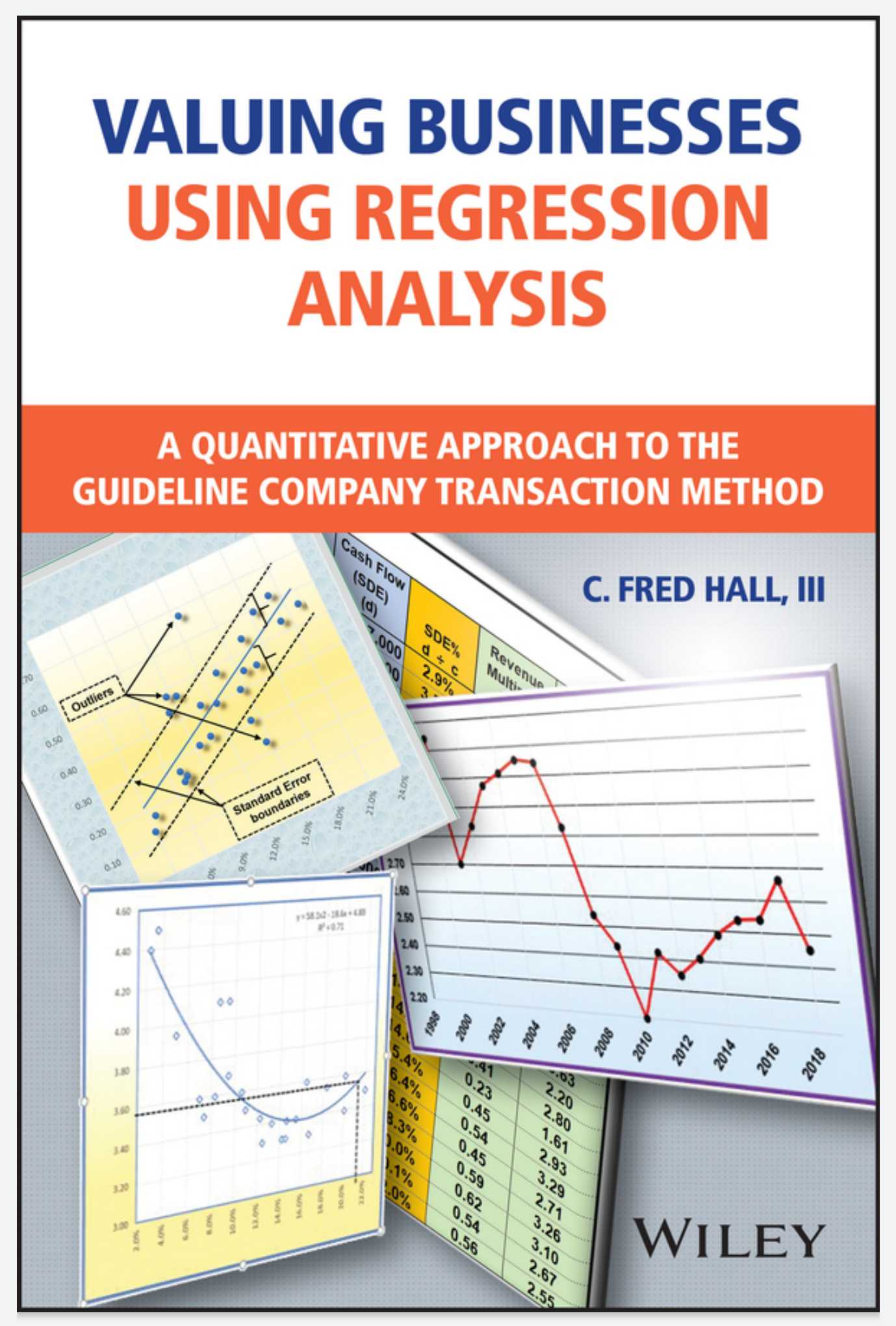# Valuing Businesses Using Regression Analysis: A Quantitative Approach to the Guideline Company Transaction Method# Valuing Businesses Using Regression Analysis: A Quantitative Approach to the Guideline Company Transaction Method

• Length: 208 pages
• Edition: 1
• Language: English
• Publisher: Wiley
• Publication Date: 2021-12-18

Description

Demystifies regression-based valuation through simple explanations, easy-to-understand charts, and time-saving bonus resources

Current methodologies using median, quartiles, or standard deviations to calculate revenue multipliers and cash flow multipliers often produce values that are wildly divergent. This forces the appraiser to choose between a very high or a very low value or consider averaging the values, opening the possibility for the appraiser to be challenged. On the surface, regression analysis appears to be the more complex, mathematical model, so many professionals shy away from using it out of fear that readers will be confused and reject the results of the appraisal. Valuing Businesses Using Regression Analysis solves this issue by breaking down regression to its simplest terms and providing easy-to-read charts and explanations that can be understood by all.

Since regression analysis does not come pre-installed in Excel, this book will show you how to enable Excel’s regression in your computer. Then you’ll learn four different Regression tools that can be used for business valuations or for forecasting in general. As an added perk, this book also comes with a template that simplifies the entire regression methodology into the click of one button. With a minimal amount of work, you can use this template to produce a compelling four-page valuation report.

• See why current valuation methodologies can be wildly inaccurate and why regression analysis is a practical and preferable alternative
• Learn how to set up Regression in Excel and use single-variable linear regression to predict revenue and cash flow multipliers
• Walk through the process for conducting more advanced analyses, including curved regression with outliers and multiple variable regression